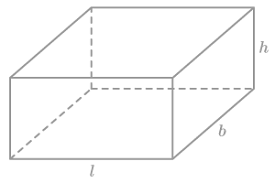# Haskell Program to Find the Surface area and Volume of Cuboid

This tutorial discusses writing a program to find the Surface area and volume of a cuboid in the Haskell programming language.

A cuboid is a solid shape that has six rectangular faces. A cuboid has three dimensions length, breadth, and height. The surface area of a cuboid is the total area of the six rectangular faces i.e 2*length*breadth + 2*length*height + 2*breadth*height. The volume of the cuboid is length*breadth*height. As volume is area*height.In this tutorial we see,

• Program to find the surface area of a cuboid.
• Program to find the volume of a cuboid.

## Algorithm Steps

• Take input or initialize the variables.
• Implement the program logic to compute the surface area and volume of the cuboid.
• Print or display the surface area and volume.

## Example 1

Program to find the surface area of a cuboid.

-- function declaration
surfaceArea :: Float->Float->Float->Float
-- function definition
surfaceArea a b h = 2*a*b + 2*a*h + 2*b*h
main = do
-- declaring and initializing variables
let a = 5
let b = 4
let h = 3
-- computing the surface area
let area = surfaceArea a b h
-- printing the surface area
print ("The surface area of a cuboid with length, breadth, and height as " ++ show a ++ ", "++show b ++ ", and " ++ show h ++" is:")
print (area)


## Output

"The surface area of a cuboid with length, breadth, and height as 5.0, 4.0, and 3.0 is:"
94.0


In the above program, we declared a function surfaceArea as such it takes three floats as arguments and returns a float. In its function definition, three arguments are accepted a, b, and h. Where a, b, and h are length, breadth, and height respectively. The surface area is computed and returned. In the main function, three variables for length, breadth, and height of the cuboid a, b, and c are declared and initialized with values 5, 4, and 3 respectively. The function surfaceArea is invoked with these initialized variables as arguments. As this function returns the surfaceArea of the cuboid, the returned output is loaded into a variable area. Finally, the surface area of the cuboid is printed with the print function.

Note − The function show takes the argument of a number and returns the parsed string of a number. “++” is an operator to concatenate strings in Haskell.

## Example 2

Program to find the volume of a cuboid.

-- function declaration
volume :: Float->Float->Float->Float
-- function definition
volume a b h = a*b*h
main = do
-- declaring and initializing variables
let a = 5
let b = 4
let h = 3
-- computing the volume
let vol = volume a b h
-- printing the volume
print ("The volume of a cuboid with length, breadth, and height as " ++ show a ++ ", "++show b ++ ", and " ++ show h ++" is:")
print (vol)


## Output

"The volume of a cuboid with length, breadth, and height as 5.0, 4.0, and 3.0 is:"
60.0


In the above program, we declared a function volume as such it takes three floats as arguments and returns a float. In its function definition, three arguments are accepted a, b, and h. Where a, b, and h are length, breadth, and height respectively. The volume is computed and returned. In the main function, three variables for length, breadth, and height of the cuboid a, b, and c are declared and initialized with values 5, 4, and 3 respectively. The function volume is invoked with these initialized variables as arguments. As this function returns the volume of the cuboid, the returned output is loaded into a variable vol. Finally, the surface area of the cuboid is printed with the print function.

## Conclusion

In this tutorial, we discussed implementing programs to find the surface area and volume of the cuboid in the Haskell programming language.